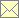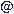PIV Contest Test Suite II (Resolution)webmasternambisde 25.6.2018

Test Suite II (Resolution)

Description

In test suite II the spatial resolution is investigated. 200 image pairs with 384×384 pixel size are generated numerically. The displacement within the light sheet is random with pixel resolution, the out-of-plane displacement is 0.25 the light sheet thickness, the light-sheet has a top-hat profile and the particle density is 0.02 per pixel. The particle images are Airy disc functions with a random intensity between 0 and 1 and a diameter of 3 pixels given by the first zero value of the Airy disc function. The intensity is integrated uniformly over the square pixel areas with fill factor 1 and intensities from different particles are linearly superimposed. No noise is added. The images are quantised with 8 bit resolution and saved in PNG grayscale format. For all images the simulated displacement is also given.

Images

The images and the simulated displacements can either be downloaded or generated on-the-fly by the simulation program. Pre-compiled executables are available for Windows and Mac OS X (Intel processor). The Pascal source is also available. To compile it, the Free Pascal compiler is recommended.

Name Convention

The images are named as ts2_xxxa.png and ts2_xxxb.png, where xxx is a number between 000 and 199 for the 200 realizations and a and b for the first and the second exposure respectively. The appropriate simulated displacements are given in the files named ts2_xxx.xyuv giving a list with four values for each pixel center position, with the coordinates x and y in pixels and the displacements u and v in pixels in x and y direction respectively. The coordinate system has its origin in the lower left corner of the lower left pixel.

Image Processing

Please, load the images or the appropriate generating programs and derive the displacement fields between the two exposures of the image pairs in pixels. Please, send back the displacement fields in text files named according to the name of the image pairs (e.g. ts2_000.xyuv for the displacement between ts2_000a.png and ts2_000b.png). Please, give the obtained vectors as a table of comma separated values (or any other delimiter) with four columns, namely the coordinates x and y and the two displacements u and v in x and y direction respectively, all in units of pixels. The coordinate system has its origin in the lower left corner of the lower left pixel. Please, list only validated vectors, since all given values are counted without a validation indicator. Non validated vectors can be skipped.

Positions and resolution of the derived vector fields can be chosen without restrictions. However, to achieve good performance grades in the test cases for spatial resolution the vector density should be chosen properly.

Important! Please use the same algorithm and the same parameters for the images from the different test suits even if normally you would change settings according to the flow field characteristics. The tests assess different statistical quantities of the PIV algorithm. The results of the individual tests then are combined to characterize the behaviour of a particular PIV processing algorithm with the particular settings. If you want to show the effect of different options of your algorithm, please, process all test suits with the different options and send back the results separately.

Analysis

From the spatially resolved displacements an inner region of 256×256 pixel is selected for comparison. In this region two matrices are reconstructed by spline interpolation with pixel resolution for both, the estimated displacements and the reference displacement field. Based on the Fourier transforms, a two-dimensional coherence function is derived, which then is decomposed into frequency response functions in x and y direction respectively.

Program

The analyzing program reads the results from the image processing and the appropriate reference displacements. From both an inner region of 256×256 pixel is selected for comparison. In this region two matrices are reconstructed by spline interpolation with pixel resolution for both, the estimated displacements and the reference displacement field. Based on the Fourier transforms, a two-dimensional coherence function is derived, which then is decomposed into frequency response functions in x and y direction respectively. In the frequency response plot both components (u and v displacements in x and y direction respectively) are merged. To estimate the error vs. the bandwidth, the displacement components are investigated separately. The bandwidth is estimated as the frequency with half the frequency response at zero frequency. The error is estimated as the RMS value of the reference displacements times the deviation of the frequency response at zero frequency from unity. The program can be called in a shell by

`ts2_contest imagedirectory prefixdirectory Algorithm1 Algorithm2 Algorithm3 ...`

where imagedirectory is the directory with the images and the reference displacement files, prefixdirectory is the directory with the subdirectories Algorithm1 etc., where Algorithm1 is the name of the first algorithm, which will be used as an identifier in the generated diagrams. For exsample ts2_contest ..\images ..\ AnaPIV.I AnaPIV.II will look in the directory ..\images for the reference displacement files and in ..\AnaPIV.I and ..\AnaPIV.II for the estimated displacements of the algorithms AnaPIV.I and AnaPIV.II respectively. If the prefix is not necessary (algorithm directories in the present directory) .\ can be used as the prefix to use the present directory as the root of the algorithm directories. The program generates the data files and an appropriate gnuplot file for visualization.

Pre-compiled executables are available for Windows and Mac OS X (Intel processor). The Pascal source is also available. To compile it, the Free Pascal compiler is recommended.

Contest Results

Frequency Response

response as a function of the spatial frequency at an out-of-plane displacement of 25% of the light sheet thickness, random displacement, light sheet profile: top-hat, particle density: 0.02 particles per pixel. For details of the PIV algorithms follow the link on the diagram.Error vs. Bandwidth

error and bandwidth (frequency yielding a response of 0.5) at an out-of-plane displacement of 25% of the light sheet thickness, random displacement, light sheet profile: top-hat, particle density: 0.02 particles per pixel. For details of the PIV algorithms follow the link on the diagram.